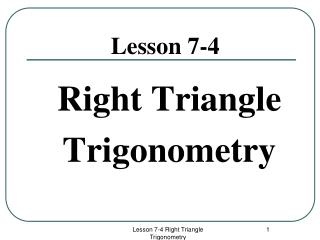Download PresentationLesson 7-4

# Lesson 7-4

Download Presentation## Lesson 7-4

- - - - - - - - - - - - - - - - - - - - - - - - - - - E N D - - - - - - - - - - - - - - - - - - - - - - - - - - -
##### Presentation Transcript

1. Lesson 7-4 Right Triangle Trigonometry Lesson 7-4 Right Triangle Trigonometry

2. In right triangles : • The segment across from the right angle ( ) is labeled the hypotenuse “Hyp.”. • The “angle of perspective” determines how to label the sides. • Segment opposite from the Angle of Perspective( ) is labeled “Opp.” • Segment adjacent to (next to) the Angle of Perspective ( ) is labeled “Adj.”. * The angle of Perspective is never the right angle. Hyp. Opp. Angle of Perspective Adj. Lesson 7-4 Right Triangle Trigonometry

3. Labeling sides depends on the Angle of Perspective If is the Angle of Perspective then …… Angle of Perspective Hyp. Adj. Opp. *”Opp.” means segment opposite from Angle of Perspective “Adj.” means segment adjacent from Angle of Perspective Lesson 7-4 Right Triangle Trigonometry

4. If the Angle of Perspective is then then Hyp Adj Hyp Opp Opp Adj Lesson 7-4 Right Triangle Trigonometry

5. Trigonometry Ratios If is the Angle of Perspective then …... Sin = Cos = tan = Hyp Opp Adj Angle of Perspective Lesson 7-4 Right Triangle Trigonometry

6. Example: Find the value of x. Step 1: Mark the “Angle of Perspective”. Step 2: Label the sides (Hyp / Opp / Adj). Step 3: Select a trigonometry ratio (sin/ cos / tan). Sin = Step 4: Substitute the values into the equation. Sin 25 = Step 5: Solve the equation : Change Sin 25 into a decimal. Cross multiply and solve. Hyp opp Angle of Perspective Adj x = (0.4226) (12) x = 5.07 cm = Lesson 7-4 Right Triangle Trigonometry

7. Solving Trigonometric Equations There are only three possibilities for the placement of the variable ‘x”. Sin = Sin = Sin = Sin 25 = Sin = Sin 25 = 0.4226 = Sin = 0.48 0.4226 = X = Sin (0.48) x = x = (12) (0.4226) x = 5.04 cm X = 28.6854 x = 28.4 cm Lesson 7-4 Right Triangle Trigonometry